# 1. HashMap继承体系

public class HashMap<K,V> extends AbstractMap<K,V> implements Map<K,V>, Cloneable, Serializable {}

• HashMap<K,V>：HashMap是以key-value形式存储数据的。
• extends AbstractMap<K,V>：继承了AbstractMap，大大减少了实现Map接口时需要的工作量。
• implements Map<K,V>：实现了Map，提供了所有可选的Map操作。
• implements Cloneable：表明其可以调用clone()方法来返回实例的field-for-field拷贝。
• implements Serializable：表明该类是可以序列化的。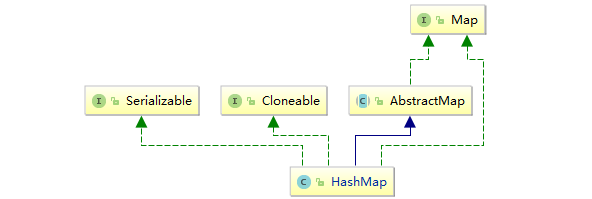# 2. HashMap的底层数据结构

HashMap的底层结构在JDK1.8 之后确定为数组+链表+红黑树。（1.7是数组+链表，这里不做分析）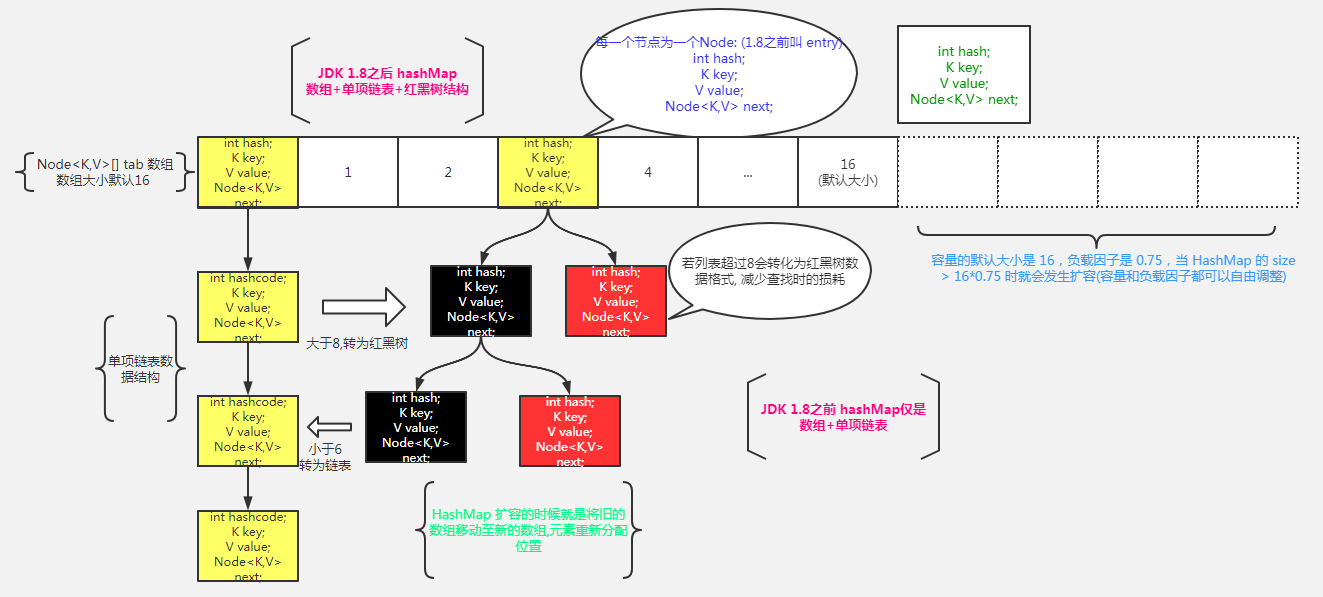通过上面结构图可以看出，其内部存储数据是封装了一种Node的数据结构，通过Node来实现结构名为table的数组。table数组中的每个元素是一个Node元素（这个Node元素可以指向下一个Node元素从而形成链表，当链表长度到达阈值后会升级为红黑树），table数组的每个位置称为桶，比如talbe 称为一个桶。

# 3. HashMap核心属性

## 3.1 静态常量

    /**
* 默认的初始容量，必须是二的次方
*/
static final int DEFAULT_INITIAL_CAPACITY = 1 << 4; // aka 16

/**
* 最大容量，当通过构造函数隐式指定了一个大于MAXIMUM_CAPACITY的时候使用
*/
static final int MAXIMUM_CAPACITY = 1 << 30;

/**
* 加载因子，当构造函数没有指定加载因子的时候的默认值的时候使用
*/
static final float DEFAULT_LOAD_FACTOR = 0.75f;

/**
* TREEIFY_THRESHOLD为当一个bin从list转化为tree的阈值，当一个bin中元素的总元素最低超过这个值的时候，bin才被转化为tree；
* 为了满足转化为简单bin时的要求，TREEIFY_THRESHOLD必须比2大而且比8要小
*/
static final int TREEIFY_THRESHOLD = 8;

/**
* bin反tree化时的最大值，应该比TREEIFY_THRESHOLD要小，
* 为了在移除元素的时候能检测到移除动作，UNTREEIFY_THRESHOLD必须至少为6
*/
static final int UNTREEIFY_THRESHOLD = 6;

/**
* 树化的另外一个阈值，table的长度的最小值为64。为了避免扩容和树型结构化阈值之间的冲突，MIN_TREEIFY_CAPACITY 应该最小是 4 * TREEIFY_THRESHOLD
*/
static final int MIN_TREEIFY_CAPACITY = 64;

## 3.2 成员变量

    /**
* table，第一次被使用的时候才进行加载
*/
transient Node<K,V>[] table;

/**
* 键值对缓存，它们的映射关系集合保存在entrySet中。即使Key在外部修改导致hashCode变化，缓存中还可以找到映射关系
*/
transient Set<Map.Entry<K,V>> entrySet;

/**
* table中 key-value 元素的个数
*/
transient int size;

/**
* HashMap在结构上被修改的次数，结构上被修改是指那些改变HashMap中映射的数量或者以其他方式修改其内部结构的次数（例如，rehash）。
* 此字段用于使HashMap集合视图上的迭代器快速失败。
*/
transient int modCount;

/**
* 下一次resize扩容阈值，当前table中的元素超过此值时，触发扩容
* threshold = capacity * load factor
*/
int threshold;

/**
* 负载因子
* @serial
*/
final float loadFactor;

# 4. HashMap构造方法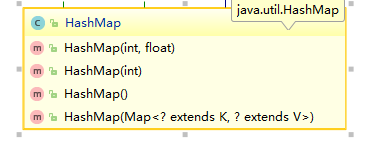public HashMap(int initialCapacity, float loadFactor) {
//初始容量值校验 范围：0 <= cap <= MAXIMUM_CAPACITY
if (initialCapacity < 0)
throw new IllegalArgumentException("Illegal initial capacity: " +
initialCapacity);
if (initialCapacity > MAXIMUM_CAPACITY)
initialCapacity = MAXIMUM_CAPACITY;
throw new IllegalArgumentException("Illegal load factor: " +
//返回一个2的次方数（大于等于当前cap）
this.threshold = tableSizeFor(initialCapacity);
}

  /**
* Returns a power of two size for the given target capacity.
*
* 1.返回一个大于等于当前值cap的一个的数字，并且这个数字一定是2的次方数
* 假如cap为10，那么n= 9 = 0b1001
* 0b1001 | 0b0100 = 0b1101
* 0b1101 | 0b0011 = 0b1111
* 0b1111 | 0b0011 = 0b1111
* ......
* .....
* n = 0b1111 = 15
*
* 2.这里的cap必须要减1，如果不减，并且如果传入的cap为16，那么算出来的值为32
*
* 3.这个方法就是为了把最高位1的后面都变为1
* 0001 1101 1100 -> 0001 1111 1111 -> +1 -> 0010 1111 1111
*/
static final int tableSizeFor(int cap) {
int n = cap - 1;
n |= n >>> 1;
n |= n >>> 2;
n |= n >>> 4;
n |= n >>> 8;
n |= n >>> 16;
return (n < 0) ? 1 : (n >= MAXIMUM_CAPACITY) ? MAXIMUM_CAPACITY : n + 1;
}

# 5. put方法解析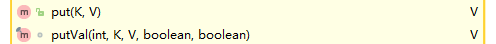put方法实际上也是调用的putVal方法，其在调用putVal方法之前有一个 扰动计算hash值 的操作，目的使降低碰撞的概率。

    /**
* 返回先前key对应的value值（如果value为null，也返回null），如果先前不存在这个key，那么返回的就是null；
*/
public V put(K key, V value) {
return putVal(hash(key), key, value, false, true);
}
/**
* 在往haspmap中插入一个元素的时候，由元素的hashcode经过一个扰动函数之后再与table的长度进行与运算才找到插入位置，下面的这个hash()方法就是所谓的扰动函数
* 作用：让key的hashCode值的高16位参与运算,hash()方法返回的值的低十六位是有hashCode的高低16位共同的特征的
* 举例
* hashCode = 0b 0010 0101 1010 1100  0011 1111 0010 1110
*
*     0b 0010 0101 1010 1100  0011 1111 0010 1110  ^
*     0b 0000 0000 0000 0000  0010 0101 1010 1100
*     0b 0010 0101 1010 1100  0001 1010 1000 0010
*/
static final int hash(Object key) {
int h;
return (key == null) ? 0 : (h = key.hashCode()) ^ (h >>> 16);
}

putVal方法

final V putVal(int hash, K key, V value, boolean onlyIfAbsent,
boolean evict) {
//tab:引用当前HashMap的散列表
//p:表示当前散列表的元素
//n:当前散列表数组的长度
//i:表示路由寻址结果
Node<K,V>[] tab; Node<K,V> p; int n, i;

//延迟初始化逻辑，第一次调用putVal时会初始化HashMap对象中的最耗费内存的散列表
if ((tab = table) == null || (n = tab.length) == 0)
n = (tab = resize()).length;

//1. 最简单的情况：寻址找到的桶位 刚好是null，直接将k-v => node 扔进去
if ((p = tab[i = (n - 1) & hash]) == null)
tab[i] = newNode(hash, key, value, null);

else {
//e:不为null的话，找到一个与当前要插入的key-value 一致的key的元素
//k:表示临时的一个key
Node<K,V> e; K k;

//2. 表示该桶位中的第一个元素与你当前插入的node元素的key一致，表示后序要进行替换操作
if (p.hash == hash &&
((k = p.key) == key || (key != null && key.equals(k))))
e = p;

//3. 表示当前桶位已经树化了,需要执行放进红黑树的逻辑
else if (p instanceof TreeNode)
e = ((TreeNode<K,V>)p).putTreeVal(this, tab, hash, key, value);

//4. 当前桶位是一个链表
else {
for (int binCount = 0; ; ++binCount) {
//4.1 条件成立的话，说明迭代到最后一个元素了，也没有找到一个与你要插入的node一致的元素，即需要放入当前链表的末尾
if ((e = p.next) == null) {
p.next = newNode(hash, key, value, null);
//新增玩元素后，当前链表长度达到树化标准（8），需要进行树化
if (binCount >= TREEIFY_THRESHOLD - 1) // -1 for 1st
//链表转红黑树
treeifyBin(tab, hash);
break;
}

//4.2 条件成立的话，说明找到了相同key的node元素，需要进行替换操作
if (e.hash == hash &&
((k = e.key) == key || (key != null && key.equals(k))))
break;
p = e;
}
}
//条件成立说明，找到了与要插入的key一致的node元素，进行替换
if (e != null) { // existing mapping for key
V oldValue = e.value;
if (!onlyIfAbsent || oldValue == null)
e.value = value;
afterNodeAccess(e);
return oldValue;
}
}

//nodeCount表示散列表table结构的修改次数，替换Node元素的value不算
++modCount;
//插入新元素，size自增，如果自增后的值大于阈值，触发扩容
if (++size > threshold)
resize();
afterNodeInsertion(evict);
return null;
}

# 6. resize方法

    final Node<K,V>[] resize() {
//region 计算newCap, newThr，为扩容做准备
//oldTab:引用扩容前的哈希表
Node<K,V>[] oldTab = table;
//oldCap：扩容之前table数组的长度
int oldCap = (oldTab == null) ? 0 : oldTab.length;
//oldThr：扩容之前的扩容阈值，触发本次库容的阈值
int oldThr = threshold;
//newCap：扩容之后table数组的大小
//newThr：扩容之后，再次触发扩容的条件
int newCap, newThr = 0;

//oldCap > 0 条件成立，说明HashMap中的散列表已经初始化过了（hashmap中的散列表不是null），要进行正常的扩容操作
if (oldCap > 0) {
//扩容之前的table数组大小已经达到 最大阈值，则不扩容，且设置扩容条件为int 最大值
if (oldCap >= MAXIMUM_CAPACITY) {
threshold = Integer.MAX_VALUE;
return oldTab;
}

//判定条件：oldCap左移一位实现数值翻倍，并赋值给newCap， newCap 小于数组最大值限制 且扩容前的阈值 >= 16
//1. 满足上述条件进行翻倍扩容(假如旧的oldCap为8， < DEFAULT_INITIAL_CAPACITY，那么此条件不成立newThr将不会赋值)
else if ((newCap = oldCap << 1) < MAXIMUM_CAPACITY &&
oldCap >= DEFAULT_INITIAL_CAPACITY)
newThr = oldThr << 1; // double threshold
}

//2. oldCap == 0的情况：说明HashMap中的散列表是null
//下面几种情况都会出现oldCap == 0,oldThr > 0
// （1）public HashMap(int initialCapacity);
// （2）public HashMap(Map<? extends K, ? extends V> m);并且这个map有数据
// （3）public HashMap(int initialCapacity, float loadFactor);
else if (oldThr > 0) // initial capacity was placed in threshold
newCap = oldThr;
else {               // zero initial threshold signifies using defaults
newCap = DEFAULT_INITIAL_CAPACITY;
}
if (newThr == 0) {
float ft = (float)newCap * loadFactor;
newThr = (newCap < MAXIMUM_CAPACITY && ft < (float)MAXIMUM_CAPACITY ?
(int)ft : Integer.MAX_VALUE);
}
//endregion

threshold = newThr;
//创建出一个更长、更大的数组
@SuppressWarnings({"rawtypes","unchecked"})
Node<K,V>[] newTab = (Node<K,V>[])new Node[newCap];
table = newTab;
//1. 本次扩容前，table不为null
if (oldTab != null) {
for (int j = 0; j < oldCap; ++j) {
//当前node节点
Node<K,V> e;
//当前桶位中有数据，但是数据具体类型还不清楚（单个数据|链表|红黑树）
if ((e = oldTab[j]) != null) {
//将对应的桶位指向null，方便jvm回收
oldTab[j] = null;
//1.1 当前桶位只有一个元素，没有发生过碰撞，此时直接计算当前元素应该存放的位置，设置即可
if (e.next == null)
//存放下标计算 ： e.hash & (newCap - 1)
newTab[e.hash & (newCap - 1)] = e;

//1.2 当前桶位为红黑树
else if (e instanceof TreeNode)
((TreeNode<K,V>)e).split(this, newTab, j, oldCap);

//1.3 当前桶位为链表
else { // preserve order
//低位链表：存放在扩容之后的数组下标位置，与当前数组的下标位置一致
Node<K,V> loHead = null, loTail = null;
//高位链表：存放在扩容之后的数组下标位置，为当前数组的下标位置+扩容之前的数组的长度
Node<K,V> hiHead = null, hiTail = null;
Node<K,V> next;
do {
//比如e.hash只能为两种可能  1 1111 或者 0 1111 ， oldCap 为 10000
//将原始链表拆为 高位链和低位链
next = e.next;
if ((e.hash & oldCap) == 0) {
if (loTail == null)
else
loTail.next = e;
loTail = e;
}
else {
if (hiTail == null)
else
hiTail.next = e;
hiTail = e;
}
} while ((e = next) != null);

//1.3.1 低位链表有数据
if (loTail != null) {
loTail.next = null;
}

//1.3.2 高位链表有数据
if (hiTail != null) {
hiTail.next = null;
}
}
}
}
}
return newTab;
}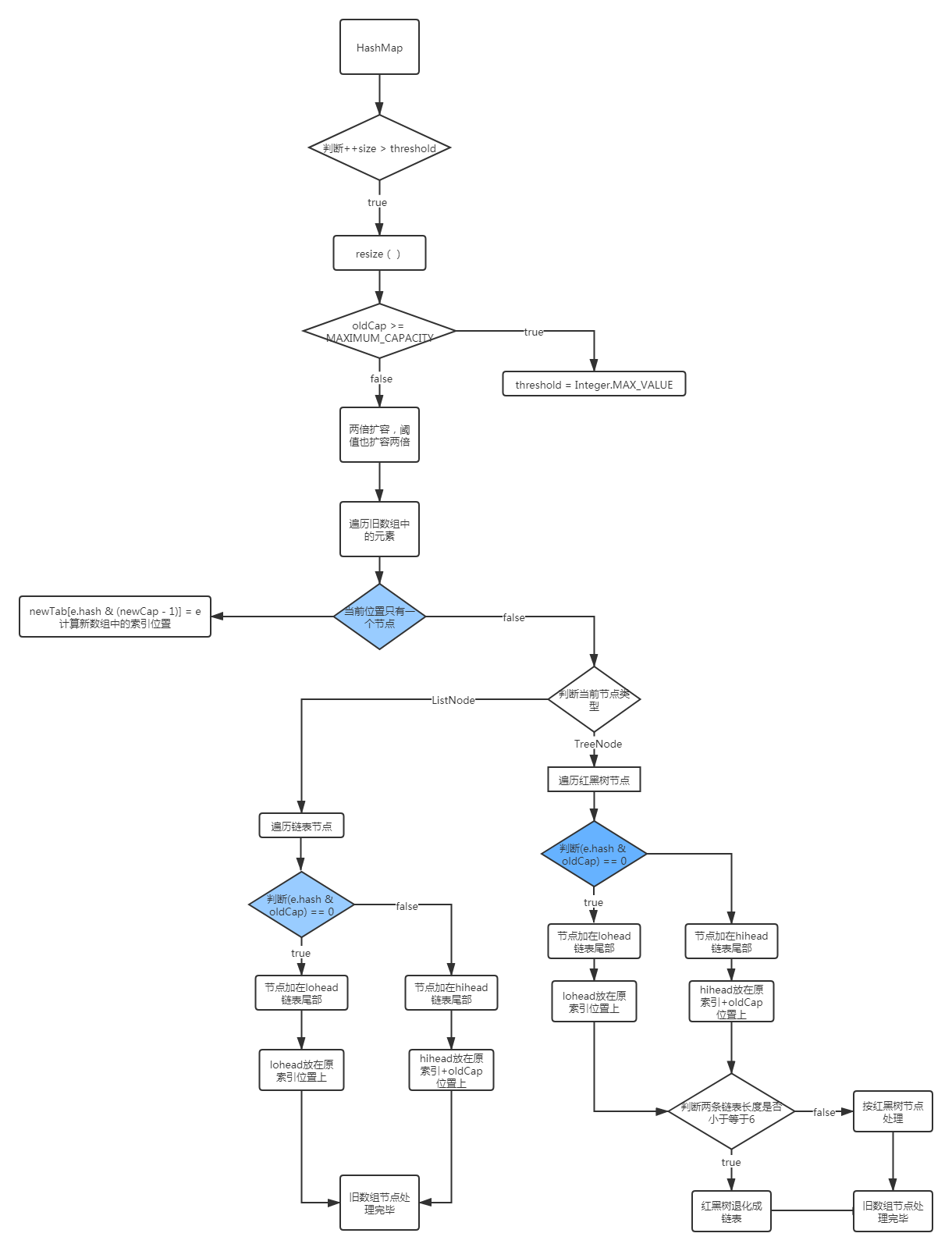# 7. remove方法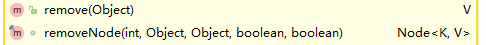remove方法也是调用的removeNode方法。

    public V remove(Object key) {
Node<K,V> e;
return (e = removeNode(hash(key), key, null, false, true)) == null ?
null : e.value;
}

/**
* Implements Map.remove and related methods
*
* @param hash hash for key
* @param key the key
* @param value the value to match if matchValue, else ignored
* @param matchValue if true only remove if value is equal
* @param movable if false do not move other nodes while removing
* @return the node, or null if none
*/
final Node<K,V> removeNode(int hash, Object key, Object value,
boolean matchValue, boolean movable) {
// tab：引用当前hashmap的table
// p：当前的node元素
// n：当前的散列表数组长度
// index：表示寻址结果
Node<K,V>[] tab; Node<K,V> p; int n, index;

//1. 如果数组table为空或key映射到的桶为空，返回null
if ((tab = table) != null && (n = tab.length) > 0 &&
(p = tab[index = (n - 1) & hash]) != null) {

// node：查找到的结果
// e：当前Node的下一个元素
Node<K,V> node = null, e; K k; V v;

//2. 桶位的头元素就是我们要找的
if (p.hash == hash &&
((k = p.key) == key || (key != null && key.equals(k))))
node = p;

//当前桶位要么是数组，要么是红黑树
else if ((e = p.next) != null) {

//3. 当前桶位已形成红黑树
if (p instanceof TreeNode)
node = ((TreeNode<K,V>)p).getTreeNode(hash, key);

//4. 当前桶位已形成链表
else {
do {
if (e.hash == hash &&
((k = e.key) == key ||
(key != null && key.equals(k)))) {
node = e;
break;
}
p = e;
} while ((e = e.next) != null);
}
}

//如果node不为null，说明按照key查找到想要删除的数据了
if (node != null && (!matchValue || (v = node.value) == value ||
(value != null && value.equals(v)))) {

//上面3.的情况，node是树节点，执行树节点移除操作
if (node instanceof TreeNode)
((TreeNode<K,V>)node).removeTreeNode(this, tab, movable);

//上面2.的情况，桶位元素执行删除
else if (node == p)
tab[index] = node.next;

//上面4.的情况，将当前元素p的下一元素 设置为 要删除元素的下一元素
else
p.next = node.next;
++modCount;
--size;
afterNodeRemoval(node);
return node;
}
}
return null;
}

# 8. get方法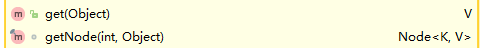get方法也是调用getNode方法，调用前有hash计算操作。

    public V get(Object key) {
Node<K,V> e;
return (e = getNode(hash(key), key)) == null ? null : e.value;
}

/**
* Implements Map.get and related methods
*
* @param hash hash for key
* @param key the key
* @return the node, or null if none
*/
final Node<K,V> getNode(int hash, Object key) {
// tab：引用当前hashmap的table
// first：桶位中的头元素
// n：table的长度
// e：是临时Node元素
// k：是key的临时变量
Node<K,V>[] tab; Node<K,V> first, e; int n; K k;

//如果哈希表为空，或key对应的桶为空，返回null
if ((tab = table) != null && (n = tab.length) > 0 &&
(first = tab[(n - 1) & hash]) != null) {

//1. 定位出来的桶位头元素即为要找的数据
if (first.hash == hash && // always check first node
((k = first.key) == key || (key != null && key.equals(k))))
return first;

//2. 当前桶位不止一个元素，其结构可能为 链表|红黑树
if ((e = first.next) != null) {

// 2.1 桶位已形成红黑树
if (first instanceof TreeNode)
return ((TreeNode<K,V>)first).getTreeNode(hash, key);

//2.2 桶位已形成链表
do {
if (e.hash == hash &&
((k = e.key) == key || (key != null && key.equals(k))))
return e;
} while ((e = e.next) != null);

}
}
return null;
}

posted @ 2020-11-02 03:52  雪山上的蒲公英  阅读(37)  评论(0编辑  收藏
/* 返回顶部代码 */